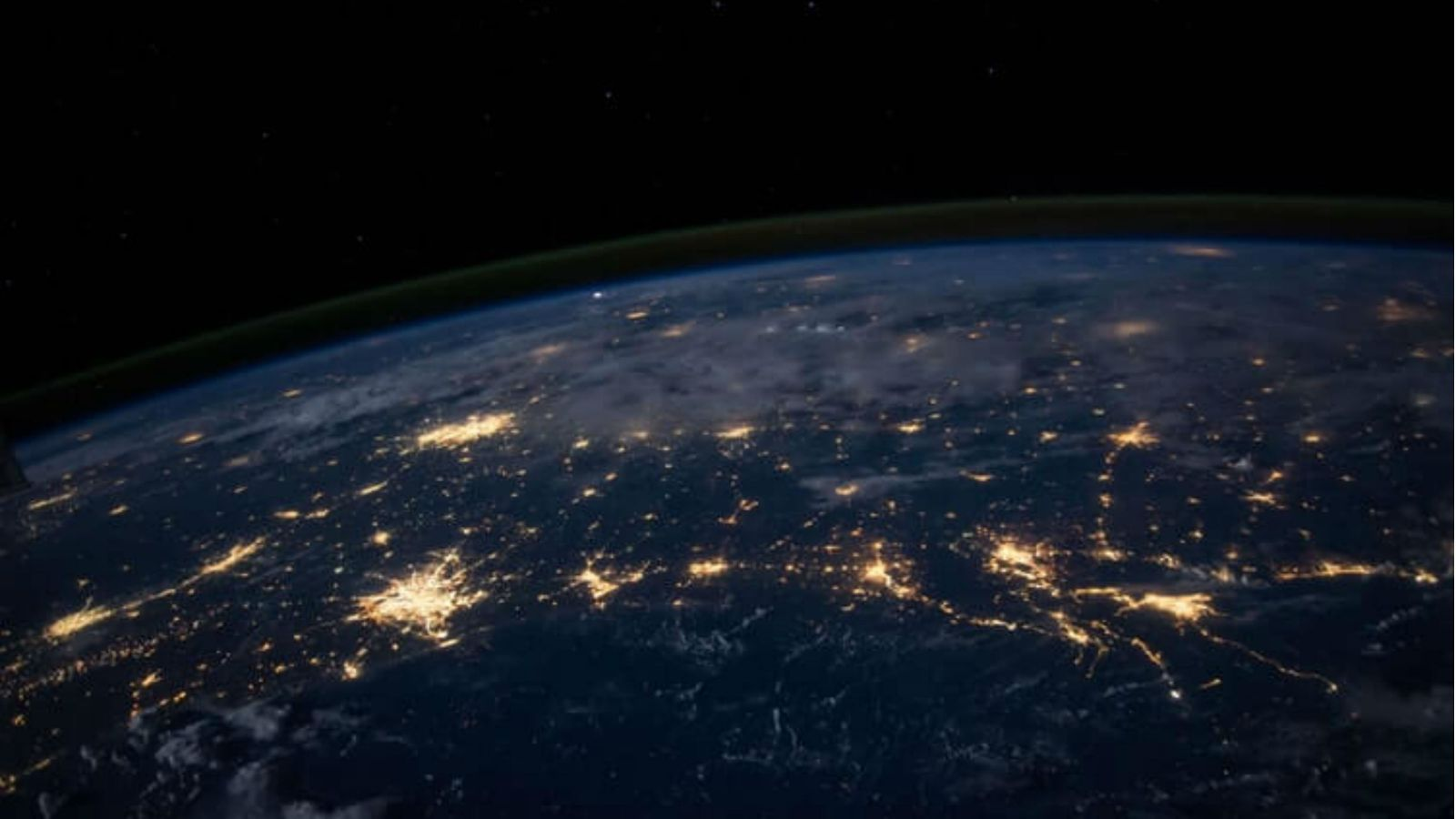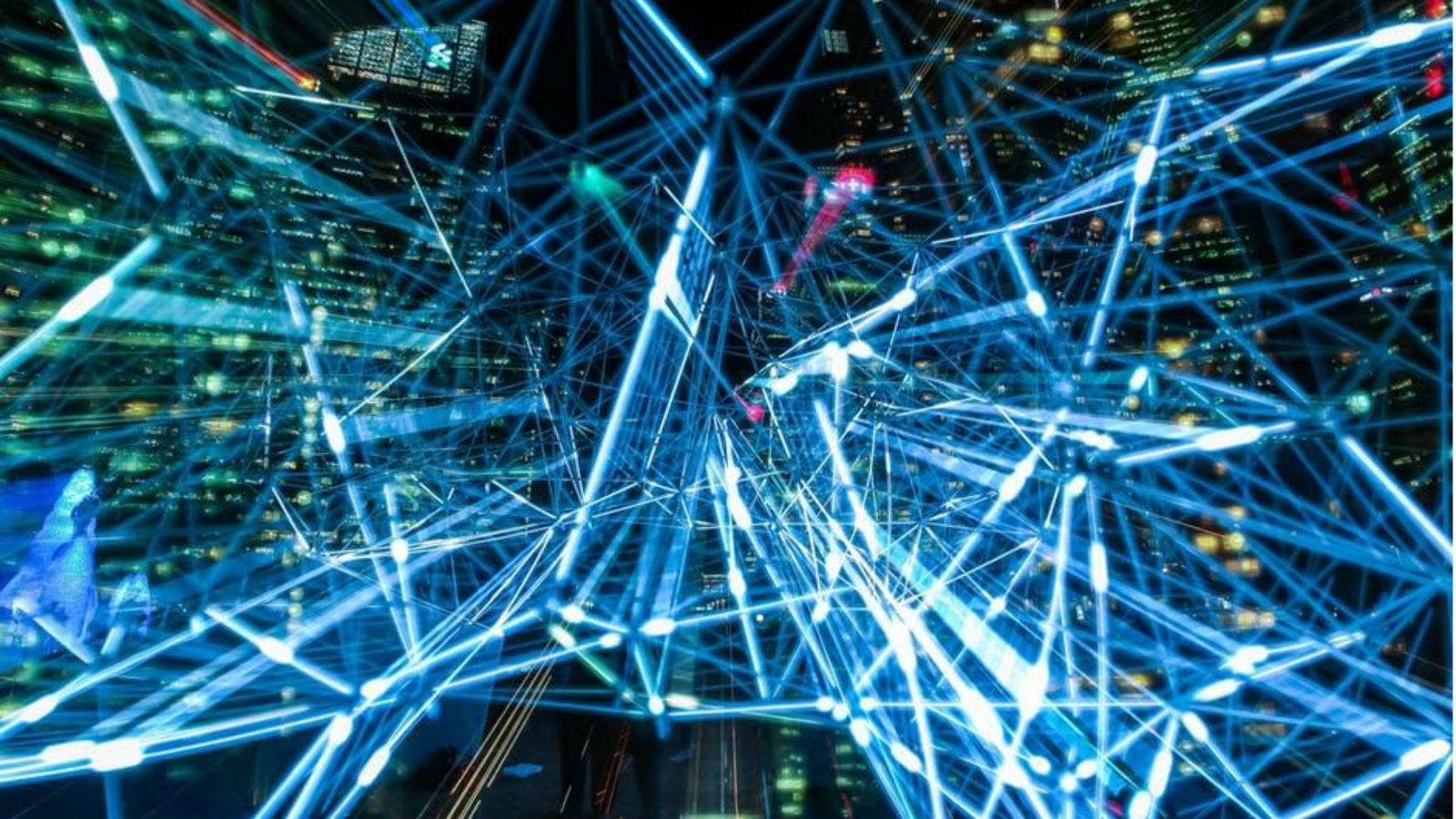# Research

Staff and postgraduate students in the School of Mathematics and Statistics collaborate in cross-disciplinary research projects across a range of subjects.

Researchers in the School of Mathematics and Statistics work in a diverse range of subject areas, in pure and applied fields. Staff and postgraduate students publish and present their work in international journals and conferences.

Staff in the School have had particular success in securing research funding from the Marsden Fund in areas such as Matroid Theory, C*-algebras, Computability, Logic, General Relativity and Stochastic Processes.

Staff and students collaborate in cross-disciplinary projects in education, biology, physics, medicine, computer science, economics and many other areas.Applied mathematics Applied mathematics ranges from theoretical physics to industrial mathematics and from geophysics to fractal geometry. The ideas involved are equally varied.Data science Data science fuses mathematics, statistics, and computer science, using aspects of all three disciplines to gain insight from data sources of all kinds.Geometry and analysis Mathematicians researching geometry and analysis are undertaking projects with postgraduate opportunities.Logic and algebra Research in the Logic and Algebra Group builds on ideas from combinatorics, mathematical logic, and general algebra.Probability and statistics Research in the Statistics Group covers a wide range of areas in both theoretical and applied probability and statistics.Statistical advice for researchers Our statistical consulting service is available for staff, students and external organisations to help with planning, implementing and analysing your research.

Name Research interests
Prof Astrid An Huef

Functional analysis, operator algebras, associative rings and algebras

Dr Becky Armstrong Functional analysis, operator algebras, associative rings and algebras
Dr Nick Brettell Matroid theory, Graph theory, Algorithms
AProf Lisa Orloff Clark Functional analysis, operator algebras, associative rings and algebras
Dr Peter Donelan

Singularities of robot kinematics, invariant theory, pseudoinverses for non-Riemannian metrics

Prof Rod Downey

Theory of computation, computability and parametrized complexity, reverse mathematics, algorithmic information theory

Prof Rob Goldblatt

Mathematical logic, general algebra

Prof Noam Greenberg

Computability theory, set theory

Dr Brendan Harding Inertial migration of particles in microfluidic devices, Fault tolerance algorithms for high performance computing, Fractal transformations

Prof John Harper

Fluid mechanics

Dr Matthew Harrison-Trainor Computability theory, Computable structure theory, model theory, differential algebra, modal logic, and probability theory.
Dr Susan Jowett Matroid Theory

Dr Byoung Du Kim

Number theory

Dr Martino Lupini Mathematical Logic, Operator algebras, Combinatorics, Dynamics
Prof Stephen Marsland Shape Analysis, diffeomorphism groups, Machine learning, complexity

Dr Dillon Mayhew

Matroid theory, graph theory, computational complexity, computability theory, finite model theory

Prof Mark McGuinness

Mathematical modelling, differential equations, industrial and geophysical mathematics, biomathematics

Dr Dimitrios Mitsotakis

Numerical analysis of partial differential equations, fluid mechanics, scientific computation and nonlinear waves

Dr Hung Pham

Banach algebras, abstract harmonic analysis

Dr Nirosha Priyardarshani
Prof Iain Raeburn

Functional analysis, operator algebras, representation theory

Dr Dan Turetsky Computability Theory

Prof Matt Visser

General relativity, quantum field theory, theoretical cosmology

Prof Geoff Whittle

Matroid theory, combinatorics

Name Research interests
Dr Ryan Admiraal Social network analysis, disease modelling

AProf Richard Arnold

Bayesian statistics, reliability, applications to geophysics (statistics of oriented objects), applications to fisheries (population models)

AProf Stefanka Chukova

Warranty Analysis, reliability

Dr Laura Dumitrescu

Data analysis, robust estimation, longitudinal data, central limit theorems
Prof Alejandro Frery Statistical Computing; Signal, Image, and Network Analysis; Data Analysis; Synthetic Aperture Radar (SAR) imagery

Dr John Haywood

Time series analysis, seasonal modelling, forecasting and statistical applications

Dr Yuichi Hirose

Data science, Statistical learning, High dimensional data analysis, Semi-parametric estimation, Survival analysis, Empirical processes.

Asymptotic methods of statistics, random processes, Statistical modelling and applications

AProf Ivy Liu

Categorical data analysis

Dr Louise McMillan model-based clustering, statistical ecology, specifically population genetics.
Dr Binh Nguyen Image Analysis and Visualization, Health Data Sciences and Informatics, Machine Learning
Dr Thuong Nguyen Asymptotic statistics

Prof Shirley Pledger

Biometrics

Dr Nokuthaba Sibanda

Bayesian inference, Spatial statistics, Bayesian modelling in fisheries and healthcare

Prof Peter Smith

Communications, signal processing

Dr Budhi Surya

Optimal stopping of Levy processes, probability applied to financial economics and actuarial science

Dr Yuan Yao Statistical learning, High-dimensional data analysis, Survival analysis, Empirical processes